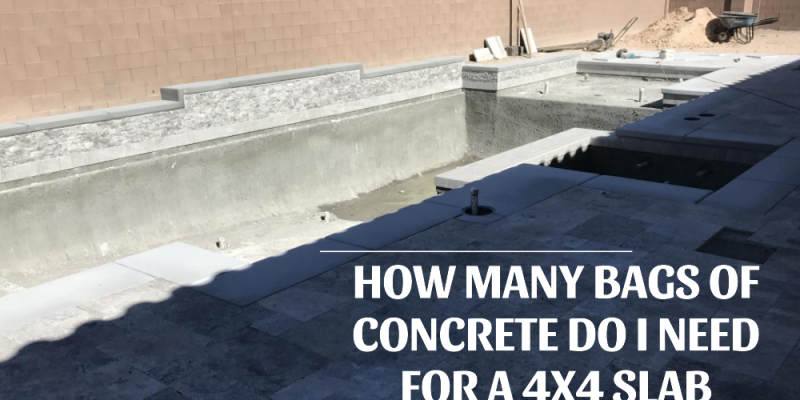Constuctioin

# How Many Bags Of Concrete Do I Need For A 4×4 SlabEmbarking on a DIY home project that involves laying a concrete slab? Understanding the amount of concrete required is fundamental to ensure efficiency and avoid unnecessary costs. This guide will walk you through the steps to determine how many bags of concrete you’ll need for a 4×4 slab.

### 1. Understanding the Basics

When we mention a 4×4 slab, it generally refers to a slab that’s 4 feet in width and 4 feet in length. However, the thickness of the slab is crucial in determining the amount of concrete needed.

### 2. The Formula

To calculate the volume of concrete required, use the formula: Volume=Length×Width×Thickness

Given our slab is 4 feet by 4 feet, you will multiply these dimensions by the thickness of the slab (in feet) to get the volume in cubic feet.

### 3. Determining the Slab Thickness

While we know our slab’s length and width, the thickness is variable. For patios or walkways, a thickness of 4 inches (which is 1/3 of a foot) is common. For driveways or slabs that need to hold more weight, you might opt for a 6-inch (or 0.5-foot) thickness.

Using the 4-inch thickness as an example: Volume=4×4×(1/3)=5.33 cubic feet

### 4. Translating Volume to Bags of Concrete

Concrete is typically sold in bags that denote the volume of concrete they can produce when mixed. Commonly, you’ll find 60-lb and 80-lb bags. An 80-lb bag of concrete typically covers around 0.6 cubic feet.

For our example: Number of 80-lb bags=5.330.6≈9

So, for a 4×4 slab that’s 4 inches thick, you’ll need approximately 9 bags of 80-lb concrete.

### 5. Factors to Consider

• Wastage: It’s always wise to purchase a little extra concrete to account for overestimation or wastage. An additional 10% is a common recommendation.
• Substrate: If the ground where you’re pouring the concrete is particularly porous, it might absorb some of the wet concrete, requiring you to use a bit more.
• Workability: If it’s your first time working with concrete, you might find the process has a steeper learning curve, potentially leading to more wastage.

### Conclusion

While calculating the amount of concrete needed for a project is a straightforward process, paying attention to the details ensures you get it right. Remember to consider factors like the slab’s purpose, the ground’s nature, and your experience level when purchasing materials. By doing so, you ensure that your 4×4 slab is both durable and cost-effective.Previous article

Next article

Constuctioin

Constuctioin

Constuctioin